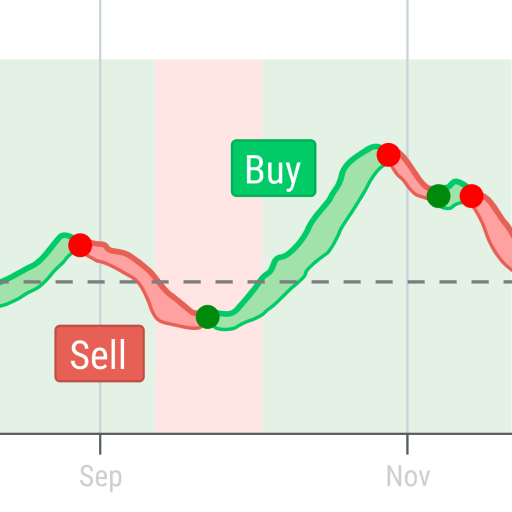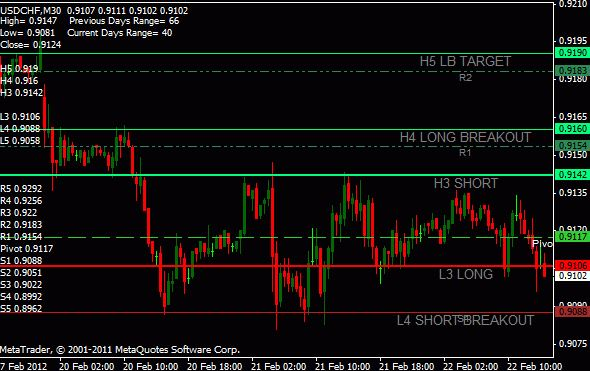buzzminer.co• Overview

This indicator displays pivot points in the chart and supports many calculation modes for Pivot Points and S/R levels

• It plots historical levels for backtesting purposes
• It allows you to select the reference timeframe
• It implements different Pivot Point calculation modes
• It implements different SR calculation modes
• It implements customizable colors and sizes

Pivot Point calculation modes

• (H+L+C)/3
• (H+L+O)/3
• (H+L+C+O)/4
• (H+L+C+C)/4
• (H+L+O+O)/4
• (H+L)/2
• (H+C)/2
• (L+C)/2

SR Levels calculation modes

Classic Formula:

• R4 = R3 + (High – Low)
• R3 = R2 + (High – Low)
• R2 = PP + (High – Low)
• R1 = (2 * PP) – Low
• S1 = (2 * PP) – High
• S2 = PP – (High – Low)
• S3 = S2 – (High – Low)
• S4 = S3 – (High – Low)

Woodie Pivot Points:

• R4 = R3 + (High – Low)
• R3 = High + 2 * (PP – Low)
• R2 = PP + (High – Low)
• R1 = (2 * PP) – Low
• S1 = (2 * PP) – High
• S2 = PP – (High – Low)
• S3 = Low – 2 * (High – PP)
• S4 = S3 – (High – Low)

Camarilla Pivot Points:

• R4 = PP + (High – Low) * 1.1/2
• R3 = PP + (High – Low) * 1.1/4
• R2 = PP + (High – Low) * 1.1/6
• R1 = PP + (High – Low) * 1.1/12
• S1 = PP – (High – Low) * 1.1/12
• S2 = PP – (High – Low) * 1.1/6
• S3 = PP – (High – Low) * 1.1/4
• S4 = PP – (High – Low) * 1.1/2
• Input parameters

When loading the indicator or EA to any chart, you will be presented with a set of options as input parameters. Don't despair if you think they are too many, because parameters are grouped into self-explanatory blocks.

PP Calculation Mode
Select the Pivot Points calculation mode desired
S/R Calculation Mode
Select the SR calculation mode desired
Timeframe
Choose the timeframe to calculate pivot points from
Mid Points
Display or hide mid points between SR levels
Color of S levels
Color of support levels
Color of R levels
Color of resistance levels
Color of P level
Color of pivot point levels
Font size
Font size for name and price labels
• ?FAQ
Does the indicator repaint?
No, the indicator never repaints.
Can I hide the historical pivot points?
No, the indicator displays historical pivot points as well for backtesting purposes.

Keep comments tidy and respectful. Avoid spam, offensive remarks, self-promotion and posting personal or payment information. The comment section is for discussion and questions only. Please note that we'll never solicit payments in the comments section.